# Centrifugal pump fault detection based on SWT and SVM

## Yu Chen1, Hongmei Liu2

1, 2School of Reliability and Systems Engineering, Beihang University, Beijing, China

2Corresponding author

Vibroengineering PROCEDIA, Vol. 19, 2018, p. 48-53. https://doi.org/10.21595/vp.2018.20165
Received 19 August 2018; accepted 27 August 2018; published 24 September 2018

33rd International Conference on Vibroengineering in Zittau, Germany, September 24-26th, 2018

Copyright © 2018 Yu Chen, et al. This is an open access article distributed under the Creative Commons Attribution License, which permits unrestricted use, distribution, and reproduction in any medium, provided the original work is properly cited.
Views 104
CrossRef Citations 0
Abstract.

Centrifugal pumps, like other rotating equipment, produce vibration signals during operation. Vibration signals often contain pump state information. Therefore, we can obtain pump state information by using appropriate signal processing methods. Synchrosqueezing wavelet transform (SWT) is a new time-frequency analysis technology. It is an algorithm for rebuilding time-frequency signals, which is similar to the empirical mode decomposition method. It can improve the time-frequency resolution of the signal compared with wavelet transform. In this paper, the SWT is used to analyze the vibration signal of centrifugal pump and extract characteristics. The data shows that the SWT can effectively extract the information of signal in time domain and frequency domain. Then we use the Support Vector Machine (SVM) to classify the features and realize the fault diagnosis of centrifugal pump. The result proves that the fault diagnosis method based on the SWT and SVM.

Keywords: centrifugal pump, fault diagnosis, synchrosqueezing wavelet transform, support vector machine.

#### 1. Introduction

Rotary machinery plays an important role in modern industrial production. Once the rotary machinery at key parts lose effectiveness, it will lead to serious consequences. Therefore, in the use of such machinery, the situation of failure needs to be taken seriously. Rotary machinery fault diagnosis technology is widely concerned in the current study. In order to realize the condition-based maintenance, it is the goal of the researcher to find out the fault as early as possible.

Centrifugal pump is a kind of rotary machinery and is widely used in many industrial fields. The fault diagnosis of centrifugal pump can effectively avoid the economic loss. There are three main types of fault diagnosis methods for centrifugal pumps: fault diagnosis based on signal, fault diagnosis based on analytic model and fault diagnosis based on expert knowledge. Based on the vibration signals of the equipment, many fault diagnosis methods have already been proposed and successfully applied in the fault diagnosis of different rotary devices. These methods use statistical analysis, frequency domain analysis or time-frequency analysis to identify and process the vibration data. According to the different characteristics of the signal, the characteristics that can represent the overall state of the vibration signal have been extracted. Finally, the classification algorithm is used to classify the characteristic so as to realize the fault diagnosis of machinery. Wang H. and Chen P. successfully monitored multiple faults of rotating machinery in real time by taking the statistical parameters of the signal, such as mean, standard deviation, kurtosis, skewness, etc., as fault characteristics and using Fuzzy Neural Network (FNN) as a classifier . Sakthivel N. R. and Sugumaran V. compared the two classification methods of decision tree and rough set, and verified by experiment on centrifugal pump . Wavelet Decomposition is used to extract the vibration signal of centrifugal pump, and Support Vector Machine is used as classifier for on-line fault diagnosis in . The vibration signal of centrifugal pump is decomposed by continuous wavelet transform and fault diagnosis is realized by support vector machine in . Given that the ensemble techniques can alleviate the generalization error and reduce the over-fitting phenomenon of the model compared with the independent algorithm, Wang Z. Y. used the selective ensemble neural network to realize fault diagnosis of rotary machinery in . Ding Y. used logistic regression to extract the vibration signal of pump, used SOFTMAX regression algorithm to classify the features in .

A fault diagnosis method based on SWT and SVM is proposed in this paper to detect the fault of centrifugal pump. Firstly, the vibration signal of centrifugal pump driven by motor is selected. The fault modes of centrifugal pump include bearing roller fault, bearing inner ring wear, bearing outer ring wear and centrifugal pump impeller wear. The time frequency analysis of vibration signal is carried out by using SWT. The time-frequency domain information of vibration signal is obtained. The frequency domain characteristics are extracted and are classified by SVM to realize the fault diagnosis of centrifugal pump.

#### 2.1. Description of SWT

Synchrosqueezing wavelet transform is a new time-frequency signal analysis method proposed by Daubeche in 2011 . By redistributing the wavelet coefficients, the signal energy in the spectrum is gathered near the real frequency, which improves the resolution of the signal in time-frequency.

For $x\left(t\right)$, its Fourier transform can be expressed as:

(1)
$x\left(w\right)=\int x\left(t\right){e}^{-jwt}dt,$

where the $w$ represents the angular frequency. The reverse transformation of $w$ is:

(2)
$x\left(t\right)=\int x\left(w\right){e}^{jwt}dw.$

Thus, the signal $x\left(t\right)$ can be expressed as the sum of a set of harmonics with different frequencies, as in Eq. (3):

(3)
$x\left(t\right)=\sum {m}_{k}\left(t\right)+e\left(t\right),$

where ${m}_{k}\left(t\right)={A}_{k}\mathrm{cos}\left({\phi }_{k}\left(t\right)\right)$ represents the $k$th harmonic component. ${A}_{k}$ represents the instantaneous amplitude of the signal, ${\phi }_{k}\left(t\right)$ represents the instantaneous phase of the $k$th harmonic component. And $e\left(t\right)$ represents the noise.

The continuous wavelet coefficients of a single harmonic signal $m\left(t\right)$ are obtained by wavelet transform as follows:

(4)
${W}_{m}\left(a,b\right)=\frac{1}{2\pi }\int {a}^{\frac{1}{2}}\stackrel{^}{m}\left(\xi \right){\stackrel{^}{\psi }}^{\mathrm{*}}\left(\alpha \xi \right){e}^{ib\xi }d\xi .$

If the main frequency of the parent wavelet is ${\omega }_{0}$, then theoretically the transformed ${W}_{m}\left(a,b\right)$ will approach $a={\omega }_{0}/\omega$ in the time-scale plane. In fact, the wavelet coefficients are divergent on the scale, which makes the time-frequency spectrum of the wavelet transform relatively fuzzy. For arbitrary $\left(a,b\right)$ and ${W}_{m}\left(a,b\right)\ne 0$, the instantaneous frequency is calculated as follows:

(5)
${\omega }_{m}\left(a,b\right)=-i{\left({W}_{m}\left(a,b\right)\right)}^{-1}\frac{\partial }{\partial b}{W}_{m}\left(a,b\right).$

The mapping of $\left(a,b\right)$ to $\left({\omega }_{m}\left(a,b\right),b\right)$ transforms the wavelet coefficients into the time-frequency plane. SWT compresses the wavelet coefficients in the interval:

Near the central frequency omega in the time-frequency plane. By processing the signal in this way, the frequency components are compressed in the frequency domain, making it easier for the frequency components to be recognized in the time-frequency plane. The discrete calculation formula of the compression wavelet coefficients is as follows:

(6)
${T}_{m}\left({\omega }_{l},b\right)=\sum _{\left|{\omega }_{m\left(a,b\right)}-{\omega }_{i}\right|\le \mathrm{\Delta }\omega /2}{W}_{m}\left(a,b\right){a}_{i}^{-3/2}{\left(\mathrm{\Delta }a\right)}_{i}.$

The continuous calculation formula is as follows:

(7)
${T}_{m}\left({\omega }_{l},b\right)={\int }_{A\left(b\right)}{W}_{m}\left(a,b\right){a}^{-3/2}da.$

#### 2.2. Description of SVM

Support Vector Machine (SVM) is a supervised learning algorithm, which is widely used in data classification and discrimination problems. SVM can effectively solve the problem of two-class classification by finding the best separation hyperplane in the feature space to maximize the interval between positive and negative samples on the training set. In order to solve the nonlinear problem, kernel function is introduced into SVM.

SVM for nonlinear classification is generally divided into two steps:

1. A nonlinear map is used to transform the data in the low-dimensional space X into a high-dimensional feature space F.

2. The linear classifier is used to realize the classification in the high-dimensional feature space F.

Consider the following data set form:

(8)
$f\left(x\right)=\sum _{i=1}^{N}{w}_{i}{\varphi }_{i}\left(x\right)+b.$

${\varphi }_{i}\left(x\right)$ is the mapping from space X to space F, but the explicit representation of the nonlinear mapping is difficult to reckon. Since the dual form is a property of SVM, the classification decision rules can be represented by the inner product of the test set and the training set:

(9)
$f\left(x\right)=\sum _{i=1}^{l}{\alpha }_{i}{y}_{i}〈\varphi \left({x}_{i}\right)\bullet \varphi \left(x\right)〉+b.$

Select a kernel function $K$, map the data to a high-dimensional space F, find the optimal separation hyperplane in space F, and distinguish the non-linear data:

(10)
$K\left(x,z\right)=〈\varphi \left(x\right)\bullet \varphi \left(z\right)〉.$

#### 3.1. Case study description

The data in the experiment is collected from the centrifugal pump in Fig. 1. Four common faults of the pump were set: bearing roller wearing, bearing inner race wearing, bearing outer race wearing and pump impeller wearing. The centrifugal pump is driven by the motor with a stable working speed of 2900 r/min. Vibration data of the centrifugal pump is obtained by accelerometers with a sampling frequency of 10.24 kHz.

Fig. 1. Centrifugal pump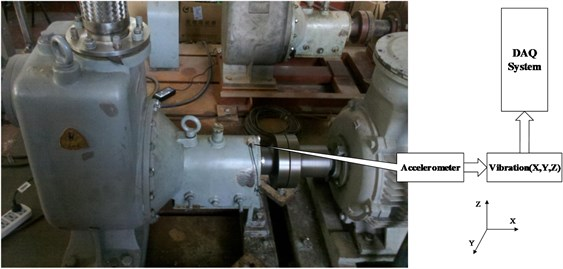#### 3.2. SWT-SVM based fault diagnosis

The collected centrifugal pump vibration signals are intercepted into a plurality of one-second long signal segments. SWT is used to analyze these signal segments. The results of frequency spectrum are in Fig. 2. It can be seen from the frequency spectrum graph that the amplitude of signal frequency domain is quite different under different fault modes.

Fig. 2. Frequency spectrum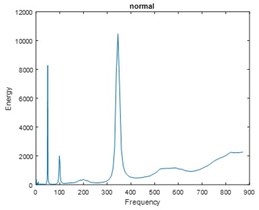a)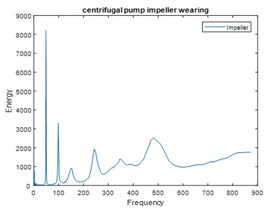b)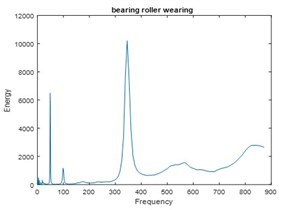c)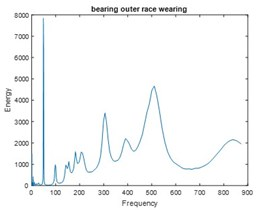d)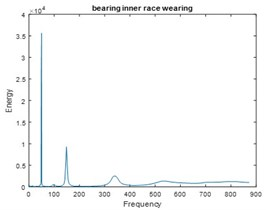e)

Given that the frequency domain characteristics of different vibration signals are quite different in the fixed frequency band, the energy of each peak part of the spectrum graph is selected as the feature. Through dimension reduction, 12000 sets of three-dimensional feature sets were obtained, including bearing roller wearing, bearing inner race wearing, bearing outer race wearing and centrifugal pump impeller wearing. There are also 2400 feature sets under normal conditions. The distribution of feature sets in three-dimensional space is shown in Fig. 3. Notably, feature sets corresponding to different states can be clearly distinguished.

Fig. 3. Distribution of feature sets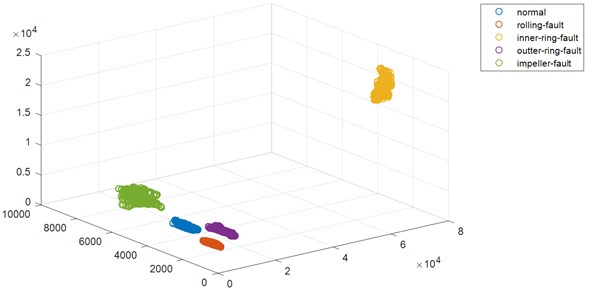SVM is chosen as classifier in this experiment. The feature set is divided into 10000 training sets and 2000 test sets, in which the training set is used to train the SVM network. After the training, we input the test sets data into classifier. Comparing the predicted values of the model output with the true values, we can get a prediction accuracy of 93.8 % for the network. The forecast result is shown in Fig. 4.

Fig. 4. The forecast result of SVM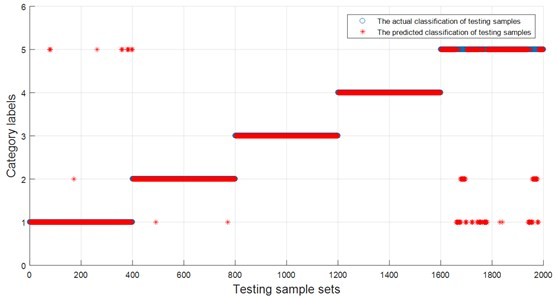#### 4. Conclusions

A fault diagnosis method of centrifugal pump based on SWT and SVM is presented in this paper. First, the vibration signal of centrifugal pump is analyzed by SWT. And the time-frequency spectrum of the signal is obtained. The SVM algorithm is used to classify different types of features. In the process of classification, some features are randomly selected to train the SVM model. When the model is trained, the feature of the unmarked fault mode is taken as the test set, which is used as the model input. And the predicted value of the test set state is used as the model output. The output of the model is a predictor of the state of the test set. Comparing the predicted value of centrifugal pump state with the actual value, we can see that the prediction accuracy of the model reaches 93.8 %. The result shows that the fault diagnosis method based on SWT and SVM can identify different fault modes with high success rate, which is helpful to realize the condition monitoring of centrifugal pump.

#### Acknowledgements

This research was supported by the National Natural Science Foundation of China (Grant Nos. 51605014, 51105019 and 51575021), the Aviation Science Fund (Grant No. 20163351018), the Technology Foundation Program of National Defense (Grant No. Z132013B002), and the Fundamental Research Funds for the Central Universities (Grant No. YWF-18-BJ-Y-159).

1. Wang H., Chen P. Sequential condition diagnosis for centrifugal pump system using fuzzy neural network. Neural Information Processing – Letters and Reviews, Vol. 11, Issue 3, 2007, p. 41-50. [Search CrossRef]
2. Sakthivel N. R., Sugumaran V., Nair B. B. Comparison of decision tree-fuzzy and rough set-fuzzy methods for fault categorization of mono-block centrifugal pump. Mechanical Systems and Signal Processing, Vol. 24, Issue 6, 2010, p. 1887-1906. [Publisher]
3. Muralidharan V., Sugumaran V., Sakthivel N. R. Wavelet Decomposition and Support Vector Machine for Fault Diagnosis of Monoblock Centrifugal Pump. Inderscience Publishers, 2011. [Publisher]
4. Kumar A., Kumar R. Time-frequency analysis and support vector machine in automatic detection of defect from vibration signal of centrifugal pump. Measurement, Vol. 108, 2017, p. 119-133. [Publisher]
5. Wang Z. Y., Lu C., Zhou B. Fault diagnosis for rotary machinery with selective ensemble neural networks. Mechanical Systems and Signal Processing, Vol. 113, 2018, p. 112-130. [Publisher]
6. Ding Y., Ma J., Tian Y. Health assessment and fault classification for hydraulic pump based on LR and softmax regression. Journal of Vibroengineering, Vol. 17, Issue 4, 2015, p. 1805-1816. [Search CrossRef]
7. Daubechies I., Lu J., Wu H. T. Synchrosqueezed wavelet transforms: a tool for empirical mode decomposition. Mathematics, arXiv:0912.2437, 2009. [Search CrossRef]